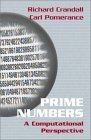# 547

This number is a prime.Prime Numbers: A Computational Perspective by Crandall and Pomerance (2002) contained exactly 547 pages.In the Disney movie The Black Hole, the crew of the Palomino escape from the parallel-universe Cygnus on Day 547.The number of primes between 6! and 7! is itself prime. 547 is the smallest odd prime to enumerate the primes between n! and (n + 1)! for any n. [Post]f(547!) + 547^3 is a prime number, where f(n!) gives the first n digits of n!. 547 is the smallest integer with this characteristic, and it is prime. [Zonta]The largest known prime number n such that the initial n digits in decimal portion of π form a prime number. [Capelle]The sum of the first 547 squares (54705470) contains 547 twice. This is the second positive integer with this property, with 5 being the first. [Gaydos]There were 547 live broadcasts of "Watch Mr. Wizard" by the time the show was canceled in 1965.The largest prime concatenated from the only three single digits d for which d!+1 are perfect squares, i.e., 5!+1 = 11^2, 4!+1 = 5^2 and 7!+1 = 71^2. [Loungrides]The Hubble Space Telescope orbits about 547 kilometers above Earth. [Harrison]Benedict of Nursia (died A.D. 547) refers to Prime by using the term "primae tempore" ("the time of First Hour") for Prime. [Jessee]

(There are 2 curios for this number that have not yet been approved by an editor.)